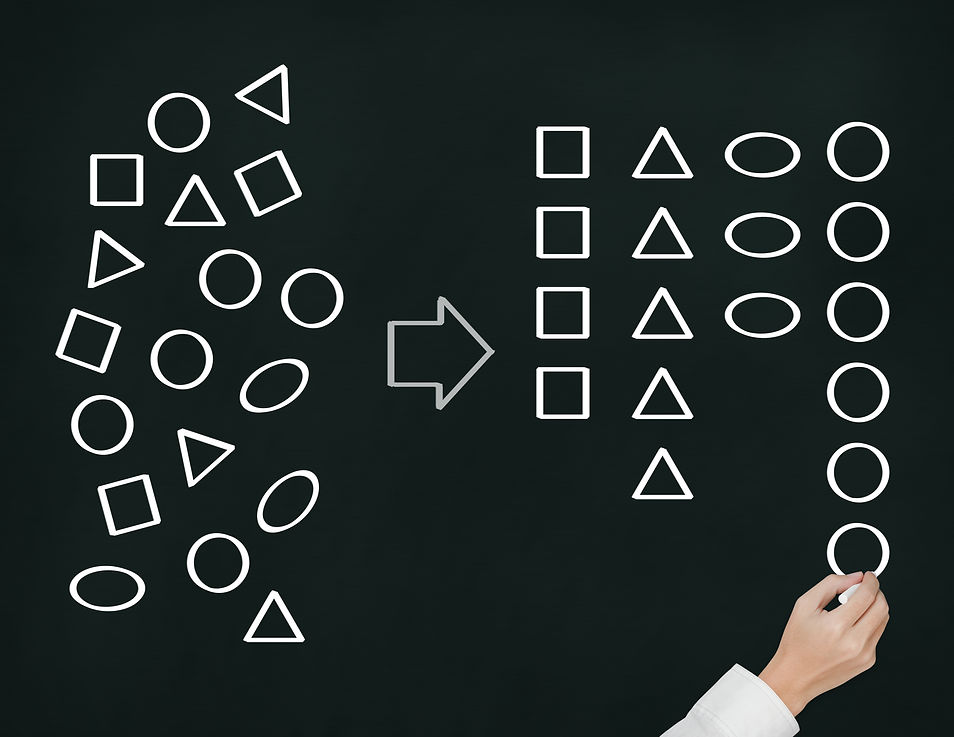## DATA ANALYSIS & PROBABILITY

Growing IQ students begin their journey with data using counting strategies (pictures and objects). As knowledge and familiarity increase, students begin to organize data into tables, utilize data to engage in more complex analysis, and calculate statistical measures (i.e., mean, median, mode, etc.).  Students also use data to answer questions that incorporate real-world problems involving probability and ratio.## 4 Categories of Stats & Probability## INTERPRETING DATA

Data can be either qualitative (non-numerical) or quantitative (numerical).  Statistics draws conclusions about data sets to give an in-depth picture of what the data is showing.## Graphs are visual representations of data that communicate detailed information concisely and clearly.  Analyzing a variety of graphs types highlights the benefits and limitations of each.## PROBABILITY

Probability describes randomness and provides an understanding of change, risk, and variation in life.  The study of probability develops mathematical reasoning skills and contributes to the conceptual knowledge of data, fractions, geometry, and more.